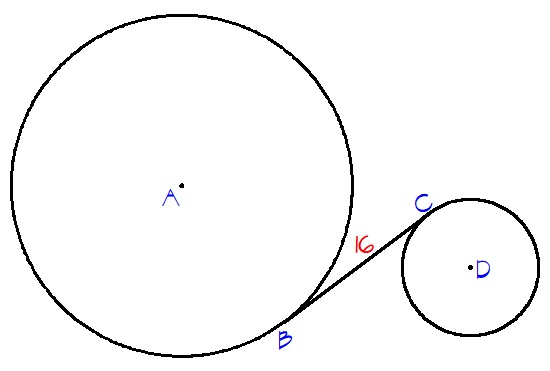# Two circles from a distance

$BC$ is tangent to both a circle with center at $A$ and a circle with center at $D$. The area of the circle with center at $A$ is $225\pi$ and the area of the circle with center at $D$ is $36\pi$.

If $BC=16$, find the distance between the centers of the two circles.×